# Solve this following

Question:

A sound source $S$ is moving along a straight track with speed $\mathrm{v}$, and is emitting sound of frequency $\mathrm{v}_{0}$ (see figure). An observer is standing at a finite distance, at the point $\mathrm{O}$, from the track. The time variation of frequency heard by the observer is best represented by :

$\left(\mathrm{t}_{0}\right.$ represents the instant when the distance between the source and observer is minimum)

1.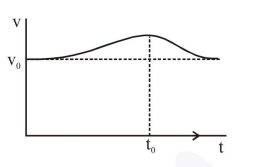2.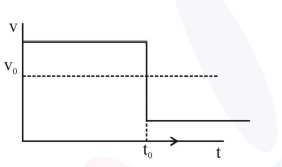3.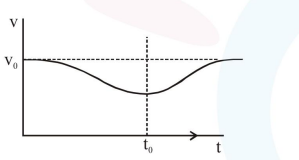4.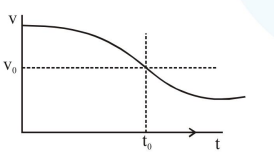Correct Option: , 4

Solution: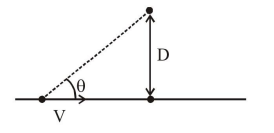$\mathrm{f}_{\text {observed }} \Rightarrow\left(\frac{\mathrm{v}_{\text {sound }}}{\mathrm{v}_{\text {sound }}-\mathrm{v} \cos \theta}\right) \mathrm{f}_{0}$

initially $\theta$ will be less $\Rightarrow \cos \theta$ more

$\therefore \mathrm{f}_{\text {observed }}$ more, then it will decrease.

$\therefore$ Ans. (4)